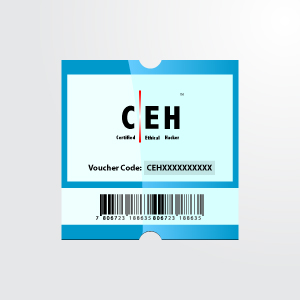Showing posts with label sum of digits. Show all posts
Showing posts with label sum of digits. Show all posts

WELCOME BACK CODERS. Here is the new blog about sum of digits in a given integer. Must check out our other blogs to get more knowledge.

This program computes the sum of digits in a given integer. The user need to enter a number then the sum of all the digits of that number will be printed.
ex:-  INPUT 31. SO the sum of 3+1 will be printed '4' .
Problem Solution
1. Take the integer as input.
2. Divide the input integer by 10, obtain its remainder and quotient.
3. Increment the new variable with the remainder got at step 2.
4. Repeat the step 2 & 3 with the quotient obtained until the quotient becomes zero.
5. Print the output and exit.

CODE
#include <stdio.h>

void main()
{
long num, temp, digit, sum = 0;

printf("Enter the number \n");
scanf("%ld", &num);
temp = num;
while (num > 0)
{
digit = num % 10;
sum = sum + digit;
num /= 10;
}
printf("Given number = %ld\n", temp);
printf("Sum of the digits %ld = %ld\n", temp, sum);
}

OUTPUT